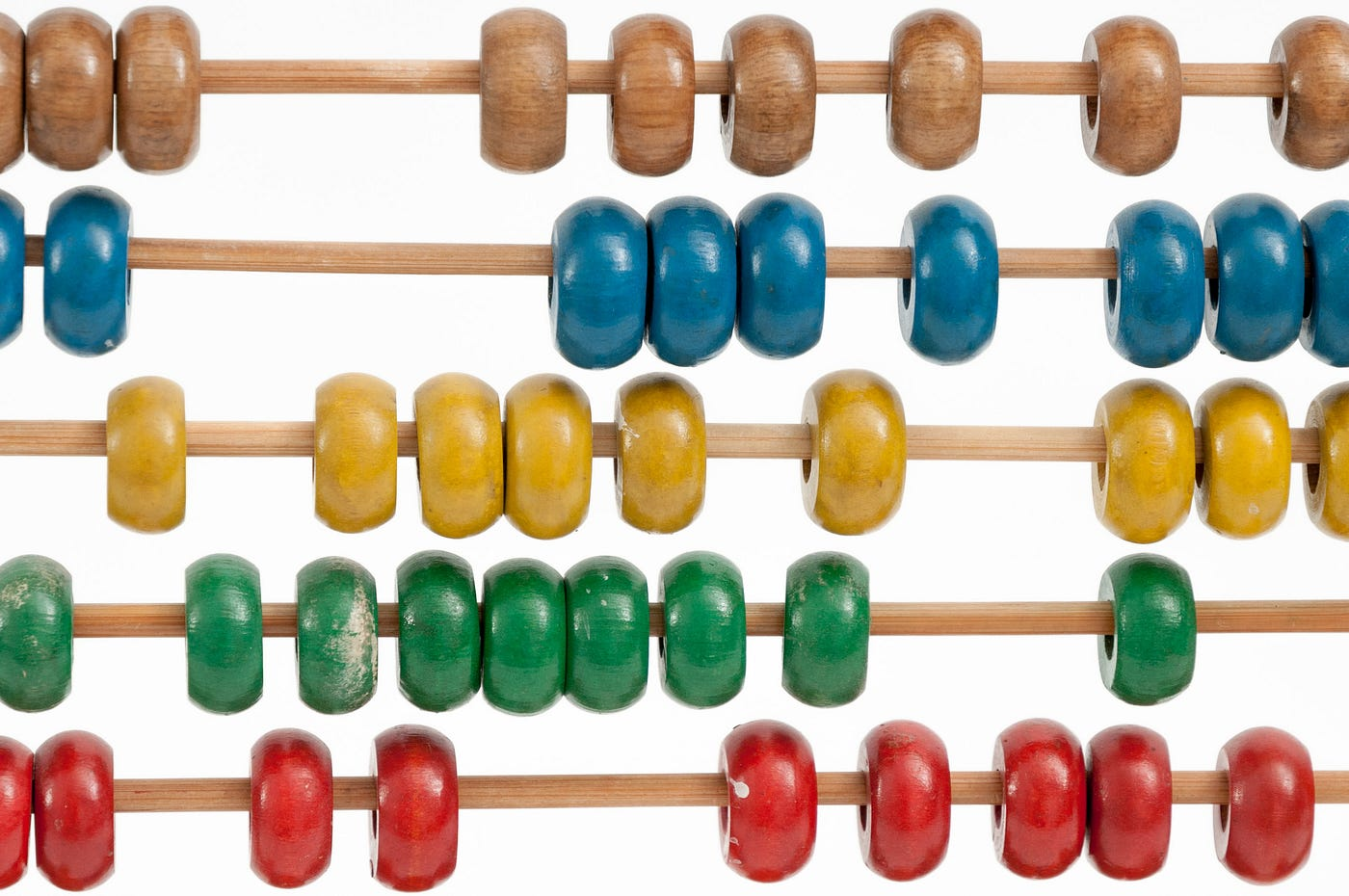# Two Sum — Day 4(Python)Photo by Crissy Jarvis on Unsplash

Today we will be looking at one of the interviewers’ favorite question for the technical coding interview.

1. Two Sum

Given an array of integers `nums` and an integer `target`, return indices of the two numbers such that they add up to `target`.

You may assume that each input would have exactly one solution, and you may not use the same element twice.

You can return the answer in any order.

Example 1:

`Input: nums = [2,7,11,15], target = 9Output: [0,1]Output: Because nums + nums == 9, we return [0, 1].`

Example 2:

`Input: nums = [3,2,4], target = 6Output: [1,2]`

Example 3:

`Input: nums = [3,3], target = 6Output: [0,1]`

Constraints:

• `2 <= nums.length <= 105`
• `-109 <= nums[i] <= 109`
• `-109 <= target <= 109`
• Only one valid answer exists.

One of the first solutions that came to my mind after reading the question is going through each element and check if the addition of any 2 elements results in the answer. If yes, we return the index of both the elements else we continue the process until we find the answer.

The code would look like the following.

`class Solution:    def twoSum(self, nums: List[int], target: int) -> List[int]:        for a in range(len(nums)):            for b in range(a+1, len(nums)):                if nums[a]+nums[b] == target:                    return [a,b]`

Complexity

Time Complexity

Considering the worst-case scenario, wherein our output is at the end of the list. In that case, we would take up O(N*(N-1)) time which is O(N²).

Space Complexity

We are not using any extra space and hence the space complexity will be O(1).

How can we make it better by improving the time complexity?

Can we use some extra space and keep track of the processed numbers?

We are required to find 2 numbers whose sum is equal to the target.

a + b = target

What if we modify the question as follows?

b = target-a

We can use a dictionary that will store the numbers that have been processed and check if the subtraction of the current number and target is available in the dictionary. If yes, then return the indices, else put the current number in the dictionary along with its index.

Let us look at the code for the above logic.

`class Solution:    def twoSum(self, nums: List[int], target: int) -> List[int]:        processed_number_dict = dict()        for a in range(len(nums)):            if (target-nums[a]) in processed_number_dict.keys():                return([a, processed_number_dict[target-nums[a]]])            else:                processed_number_dict[nums[a]] = a`

Complexity.

Time Complexity

We are traversing through the array once and hence the time complexity will be O(N)

Space Complexity

We are using a dictionary to store the elements in the array. In the worst-case scenario, if both our numbers are at the end of the array, then we will be storing N-2 numbers in our dictionary. And hence the space complexity of the above solution is O(N).

I would like to improve my writing skills so any suggestions or critiques are highly welcomed.

--

--